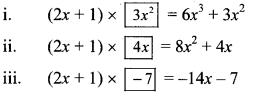# Maharashtra Board 8th Class Maths Miscellaneous Exercise 2 Solutions

Balbharti Maharashtra State Board Class 8 Maths Solutions covers the Miscellaneous Exercise 2 8th Std Maths Answers Solutions.

## Miscellaneous Exercise 2 8th Std Maths Answers

Question 1.
Questions and their alternative answers are given. Choose the correct alternative answer.
i. Find the circumference of a circle whose area is 1386 cm²? [Chapter 15]
(A) 132 cm²
(B) 132 cm
(C) 42 cm
(D) 21 cm²
Solution:
(B) 132 cm

Hint:
i. Area of the circle = πr²
1386 = $$\frac { 22 }{ 7 }$$ x r²
r² = 1386 x $$\frac { 7 }{ 22 }$$
= 63 x 7
= 441
r = √441 … [Taking square root of both sides]
= 21 cm
Circumference of the circle = 2πr
= 2 x $$\frac { 22 }{ 7 }$$ x 21
= 132 cm

ii. The side of a cube is 4 m. If it is doubled, how many times will be the volume of the new cube, as compared with the original cube? [Chapter 16]
(A) Two times
(B) Three times
(C) Four times
(D) Eight times
Solution:
(D) Eight times

Hint:
ii. Original volume = (4)³ = 64 cu.m
New side = 8 m
∴ New volume = (8)² = 512 cu.m
Now, $$\frac{\text { new volume }}{\text { original volume }}=\frac{512}{64}$$ = 8
original volume 64
∴ volume of new cube will increase 8 times as compared to the volume of original cube.

Question 2.
Pranalee was practicing for a 100 m running race. She ran 100 m distance 20 times. The time required, in seconds, for each attempt was as follows. [Chapter 11]
18, 17, 17, 16,15, 16, 15, 14,16, 15, 15, 17, 15, 16,15, 17, 16, 15, 14,15
Find the mean of the time taken for running.
Solution:∴ The mean of the time taken for running 100 m race is 15.7 seconds.

Question 3.
∆DEF and ∆LMN are congruent in the correspondence EDF ↔ LMN. Write the pairs of congruent sides and congruent angles in the correspondence. [Chapter 13]
Solution: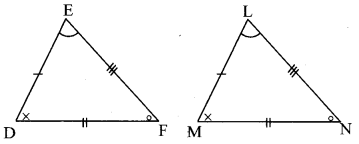∆EDF ≅ ∆LMN
∴side ED ≅ side LM
side DF ≅ side MN
side EF ≅ side LN
∠E ≅∠L
∠D ≅∠M
∠F ≅∠N

Question 4.
The cost of a machine is Rs 2,50,000. It depreciates at the rate of 4% per annum. Find the cost of the machine after three years. [Chapter 14]
Solution:
Here, P = Cost of the machine = Rs 2,50,000
R = Rate of depreciation = 4%
N = 3 Years
A = Depreciated price of the machine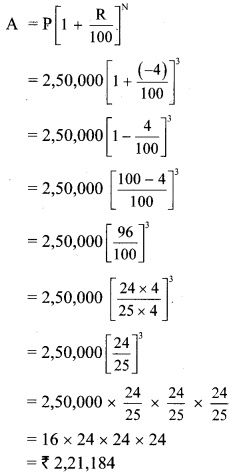∴The cost of the machine after three years will be Rs 2,21,184.

Question 5.
In ☐ABCD, side AB || side DC, seg AE ⊥ seg DC. If l(AB) = 9 cm, l(AE) = 10 cm, A(☐ABCD) = 115 cm² , find l(DC). [Chapter 15]
Solution:
Given, side AB || side DC.
∴ ☐ABCD is a trapezium.Given, l(AB) = 9 cm, l(AE) = 10 cm,
A(☐ABCD) = 115 cm²
Area of a trapezium
= $$\frac { 1 }{ 2 }$$ x sum of lengths of parallel sides x height
∴ A(☐ABCD) = $$\frac { 1 }{ 2 }$$ x [l(AB) + l(DC) x l(AE)]
∴ 115 = $$\frac { 1 }{ 2 }$$ x [9 + l(DC)] x 10
∴ $$\frac { 115 \times 2 }{ 10 }$$ = 9 + l(DC)
∴ 23 = 9 + l(DC)
∴ l(DC) = 23 – 9
∴ l(DC) = 14cm

Question 6.
The diameter and height of a cylindrical tank is 1.75 m and 3.2 m respectively. How much is the capacity of tank in litre?
[π = $$\frac { 22 }{ 7 }$$] [Chapter 16]
Solution:
Given: For cylindrical tank:
diameter (d) = 1.75 m, height (h) = 3.2 m
To Find: Capacity of tank in litre
diameter (d) = 1.75 m
= 1.75 x 100
….[∵ 1 m = 100cm]
= 175 cm
∴ radius (r) = $$=\frac{\mathrm{d}}{2}=\frac{175}{2}$$ cm
h = 3.2 cm
= 3.2 x 100
= 320 cm
Capacity of tank = Volume of the cylindrical tank
= πr²h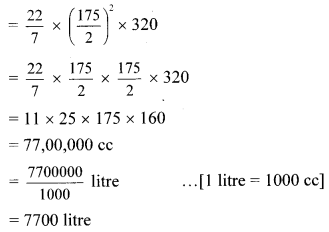∴ The capacity of the tank is 7700 litre.

Question 7.
The length of a chord of a circle is 16.8 cm, radius is 9.1 cm. Find its distance from the centre. [Chapter 17]
Solution:Let CD be the chord of the Circle with centre O.
Draw seg OP ⊥ chord CD
∴l(PD) = $$\frac { 1 }{ 2 }$$ l(CD)
…[Perpendicular drawn from the centre of a circle to its chord bisects the chord]
∴l(PD) = $$\frac { 1 }{ 2 }$$ x 16.8 …[l(CD) = 16.8cm]
∴l(PD) = 8.4 cm …(i)
∴In ∆OPD, m∠OPD = 90°
∴[l(OD)]² = [l(OP)]² + [l(PD)]² …..[Pythagoras theorem]
∴(9.1)² = [l(OP)]² + (8.4)² … [From (i) and l(OD) = 9.1 cm]
∴(9.1)² – (8.4)² = [l(OP)]²
∴(9.1 + 8.4) (9.1 – 8.4) = [l(OP)]²
…[∵ a² – b² = (a + b) (a – b)]
∴17.5 x (0.7) = [l(OP)]²
∴12.25 = [l(OP)]²
i.e., [l(OP)]² = 12.25
∴l(OP) = √12.25
…[Taking square root of both sides]
∴l(OP) = 3.5 cm
∴The distance of the chord from the centre is 3.5 cm.

Question 8.
The following tables shows the number of male and female workers, under employment guarantee scheme, in villages A, B, C and D.

 Villages A B C D No. of females 150 240 90 140 No. of males 225 160 210 110

i. Show the information by a sub-divided bar-diagram.
ii. Show the information by a percentage bar diagram. [Chapter 11]
Solution:
i.

 Villages A B C D No. of females 150 240 90 140 No. of males 225 160 210 110 Total 375 400 300 250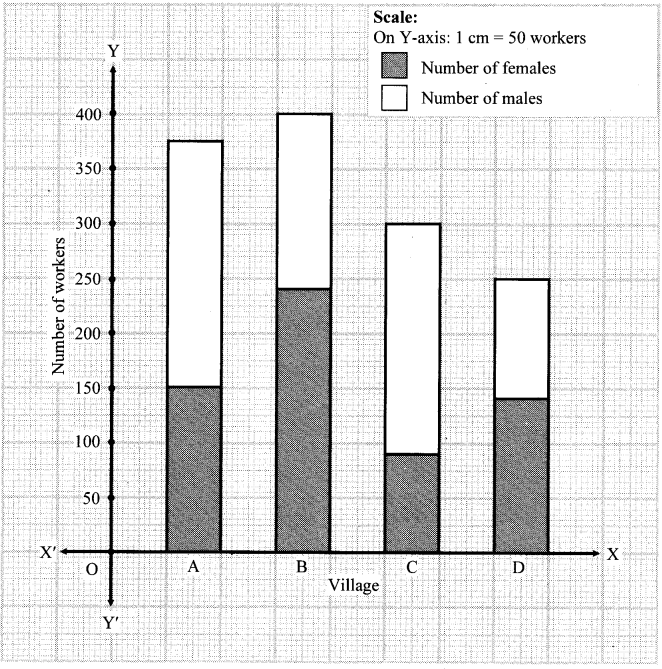ii.

 Villages A B C D No. of females 150 240 90 140 No. of males 225 160 210 110 Total 375 400 300 250 Percentage of females 40% 60% 30% 56% Percentage of males 60% 40% 70% 44%Question 9.
Solve the following equations.
i. 17 (x + 4) + 8 (x + 6) = 11 (x + 5) + 15 (x + 3)
ii. $$\frac{3 y}{2}+\frac{y+4}{4}=5-\frac{y-2}{4}$$
iii. 5(1 – 2x) = 9(1 -x)
[Chapter 12]
Solution:
i. 17 (x + 4) + 8 (x + 6) = 11 (x + 5) + 15 (x + 3)
∴ 17x + 68 + 8x + 48 = 11x + 55 + 15x + 45
∴ 17x + 8x + 68 + 48 = 11x + 15x + 55 + 45
∴ 25x + 116 = 26x + 100
∴ 25x + 116 – 116 = 26x + 100 – 116
… [Subtracting 116 from both the sides]
∴ 25x = 26x – 16
∴ 25x – 26x = 26x – 16 – 26x
… [Subtracting 26x from both the sides]
∴ -x = -16
∴ $$\frac{-x}{-1}=\frac{-16}{-1}$$
∴ x = 16

ii. $$\frac{3 y}{2}+\frac{y+4}{4}=5-\frac{y-2}{4}$$
∴ $$\frac{3 y \times 2}{2 \times 2}+\frac{y+4}{4}=5-\frac{y-2}{4}$$
∴ $$\frac{6 y}{4}+\frac{y+4}{4}=5-\frac{y-2}{4}$$
∴ $$\frac{6 y}{4} \times 4+\frac{y+4}{4} \times 4=5 \times 4-\frac{y-2}{4} \times 4$$
……[Multiplying both the sides by 4]
∴ 6y + y + 4 = 20 – (y – 2)
∴ 7y + 4 = 20 – y + 2
∴ 7y + 4 = 22 – y
∴ 7y + 4 – 4 = 22 – y – 4
…..[Subtracting 4 from both the sides]
∴ 7y = 18 – y
∴ 7y + y = 18 – y + y
…[Adding y on both the sides]
∴ 8y = 18
∴ $$\frac{8 y}{8}=\frac{18}{8}$$ … [Dividing both the sides by 8]
∴ $$y=\frac { 9 }{ 4 }$$

iii. 5(1 – 2x) = 9(1 – x)
∴ 5 – 10x = 9 – 9x
∴ 5 – 10x – 5 = 9 – 9x – 5
….[Subtracting 5 from both the sides]
∴ -10x = 4 – 9x
∴ -10x + 9x = 4 – 9x + 9x
… [Adding 9x on both the sides]
∴ -x = 4
∴ -x x (- 1) = 4 x (- 1)
… [Multiplying both the sides by – 1]
∴ x = – 4

Question 10.
Complete the activity according to the given steps.
i. Draw rhombus ABCD. Draw diagonal AC.
ii. Show the congruent parts in the figure by identical marks.
iii. State by which, test and in which correspondence ∆ADC and ∆ABC are congruent.
iv. Give reason to show ∠DCA ≅ ∠BCA, and ∠DAC ≅ ∠BAC
v. State which property of a rhombus is revealed from the above steps. [Chapter 13]
Solution:
a.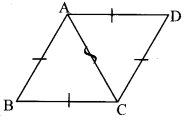side AD ≅ side AB …..[Sides of a rhombus]
side DC ≅ side BC …..[Sides of a rhombus]
side AC ≅ side AC … [Common side]
∆ADC ≅ ∆ABC … [By SSS test]
∠DCA ≅ ∠BCA …[Corresponding angles of congruent triangles]
∠DAC ≅ ∠BAC …[Corresponding angles of congruent triangles]
From the above steps, property of rhombus revealed is ‘diagonal of a rhombus bisect the opposite angles’.

Question 11.
The shape of a farm is a quadrilateral. Measurements taken of the farm, by naming its corners as P, Q, R, S in order are as follows. l(PQ) = 170 m,
l(QR) = 250 m, l(RS) = 100 m, l(PS) = 240 m, l(PR) = 260 m.
Find the area of the field in hectare (1 hectare = 10,000 sq.m). [Chapter 15]
Solution: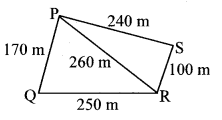Area of the field = A(∆PQR) + A(∆PSR)
In ∆PQR, a = 170 m, b = 250 m, c = 260 m
Semiperimeter of ∆PQR = sArea of the field = A(∆PQR) + A(∆PSR)
= 20400 + 12000
= 32400 sq.m
= $$\frac { 32400 }{ 10000 }$$
…[1 hectare = 10,000 sq.m]
= 3.24 hectares
∴ The area of the field is 3.24 hectares.

Question 12.
In a library, 50% of total number of books is of Marathi. The books of English are $$\frac { 1 }{ 3 }$$ of Marathi books. The books on Mathematics are 25% of the English books. The remaining 560 books are of other subjects. What is the total number of books in the library? [Chapter 12]
Solution:
Let the total number of books in the library be x
50% of total number of books is of Marathi.
Number of Marathi books = 50% of x
= $$\frac { 50 }{ 100 }x$$
= $$\frac { x }{ 2 }$$
The books of English are $$\frac { 1 }{ 3 }$$ of Marathi books.
Number of books of English = $$\frac{1}{3} \times \frac{x}{2}$$
= $$\frac { x }{ 6 }$$
The books on Mathematics are 25% of the English books.
Number of books of Mathematics
= 25% of $$\frac { x }{ 6 }$$
= $$\frac{25}{100} \times \frac{x}{6}$$
= $$\frac { x }{ 24 }$$
Since, there are 560 books of other subjects, the total number of books in the library are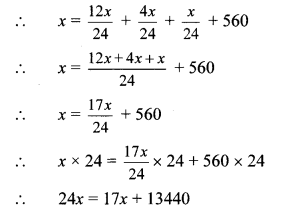∴ 24x – 17x = 17x + 13440 – 17x
∴ 7x = 13440
∴ $$\frac{7 x}{7}=\frac{13440}{7}$$
∴ x = 1920
∴ The total number of books in the library are 1920.

Question 13.
Divide the polynomial (6x³ + 11x² – 10x – 7) by the binomial (2x + 1). Write the quotient and the remainder. [Chapter 10]
Solution: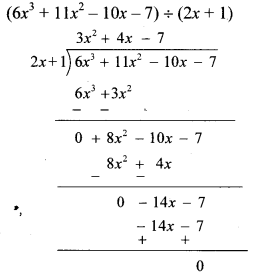∴ Quotient = 3x² + 4x – 7,
remainder = 0
Explanation: